# SSAT Middle Level Math : How to find percentage

## Example Questions

← Previous 1

### Example Question #66 : Arithmetic

A store normally sells a certain coat for $40. For the holidays it offers a sale where the new price of the coat is now$30.  What percent discount is the store selling the coat for?

Possible Answers: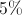discount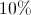discount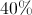discount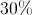discount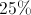discount

Correct answer:discount

Explanation:

The coat is $10 cheaper than normal.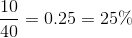### Example Question #1 : How To Find Percentage Write 132% as a fraction in simplest form. Possible Answers: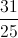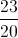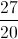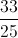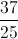Correct answer:Explanation: Write as a fraction over 100, and simplify: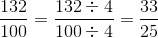### Example Question #2 : How To Find Percentage Writeas a percent. Possible Answers: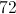%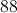%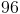%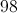%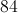% Correct answer:% Explanation: Multiply by 100, and simplify: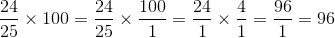The correct choice is 96%. ### Example Question #3 : How To Find Percentage What is the decimal form of 42.5%? Possible Answers: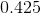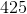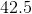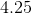Correct answer:Explanation: This question is asking you to convert the percent into a decimal. When doing this, you must move the decimal point two spaces to the left. The answer is### Example Question #1 : Percentages Marshal saves 10% of his paycheck each week. If Marshal earned$652.20 this week, approximately how much money did he save?

Possible Answers: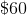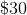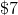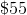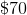Correct answer:Explanation:

The word approximately tells you that you need to estimate to get the answer. $652.20 can round up to$700 because 52.20 is more than half of 100. Since 10% is equal to the decimal 0.10, we multiply 700 by this number in order to find out what 10% of 700 is.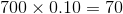The answer is### Example Question #2042 : Ssat Middle Level Quantitative (Math)

What percentage ofis?

Possible Answers: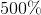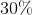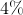Correct answer:Explanation:

To find the percentage, first write the values as a fraction, then convert the fraction's denominator to. We can write the given values as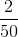, which can be converted to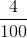by multiplying both the numerator and denominator byis equivalent to.

### Example Question #1381 : Numbers And Operations

What percent (%) of 50 is 30?

Possible Answers:

60%

30%

166%

40%

50%

Correct answer:

60%

Explanation:

The original number in this problem is 50 and you must find the percentage of 30 from 50.

Another way to view this problem is what percentage of the original 50 equals 30.

Let x equal the percentage:

50(x) = 30

50x = 30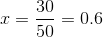In order to change the decimal 0.6 to a percentage multiply by 100:

0.6 x 100 = 60%

### Example Question #1382 : Numbers And Operations

There were an estimated 20.5 million registered cars in the United States in 1995. The 15 years later the number of registered cars was an en estimated 25.0 million. What is the percent increase in registered cars from 1995 to 2010 rounded to the nearest whole number?

Possible Answers: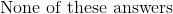Correct answer:Explanation:

The formula for the percent increase is: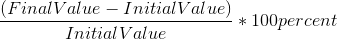Substituting the given values into the above equation: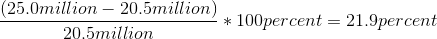After rounding, the answer is 22%

The other answers represent possible mistakes that could have been made:  18% is the mistake of dividing by the final value, not the initial value;  10% is the mistake of dividing by the sum of the final and the initial values; and 21% is the mistake of improper rounding or not doing the division to the third digit.

### Example Question #1 : How To Find Percentage

Ifout ofstudents have contact lenses, what percentage of students have contact lenses?

Possible Answers:percentpercentpercentpercentpercent

Correct answer:percent

Explanation:

When dividingby, the reduced form is: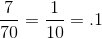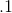is the equivalent ofpercent, which is therefore the correct answer.

### Example Question #1384 : Numbers And Operations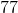is what percent of?

Possible Answers: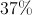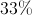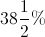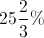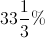Correct answer:Explanation:

Set up the proportion statement, whereis our answer, and solve: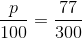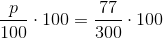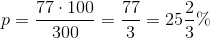← Previous 1

### All SSAT Middle Level Math Resources